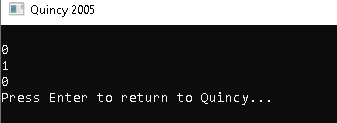# Logical Operators

#### Logical Operators

To execute logical operations, logical operators are employed. Boolean expressions are used with logical operators, which return a Boolean value. In loops and decision-making statements, logical operators are combined with conditional operators.

#### Syntax

operands_one || operands_two; operands_one && operands_two;

#### The use of logical operators

#1) Symbol for the Logical AND Operator: "&&" The AND operator returns true when both values are true. It will return false if any of the values are false.

• For example, if both A and B are true, A && B will return true; if either or both are false, it will return false.

#2) Symbol for the Logical OR Operator: "||" The OR operator returns true if any of the conditions/operands are true. When both operands are false, it will return false.

• A || B, for example, returns true if either A or B has a true value. If both A and B are false, it will return false.

#3) Symbol for the Logical NOT Operator: "!" The NOT operator reverses any condition's logical conclusion. The result is false if the condition is true; the result is true if the condition is false.

• For example, if "A||B" returns true,!(A||B) returns false, and if "A||B" returns false, it returns true.

#### Example Program:

#include <iostream> using namespace std; int main() { int a = 10; int b = 5; bool result; // AND operator result = (a == b) && (a>b); cout<<"\n"<<(result); //OR Operator result = (a == b) || (a>b); cout<<"\n"<<(result); //NOT Operator result = !((a == b) || (a>b)); cout<<"\n"<<(result); return 0; }

#### OUTPUT:#### The following is the program's output:

• Because one of the criteria, a==b, is false, the first value will return false.
• As one of the criteria, a > b, is true, the second operator will return true.
• The third operator, which is the negation of the OR result, will yield false.